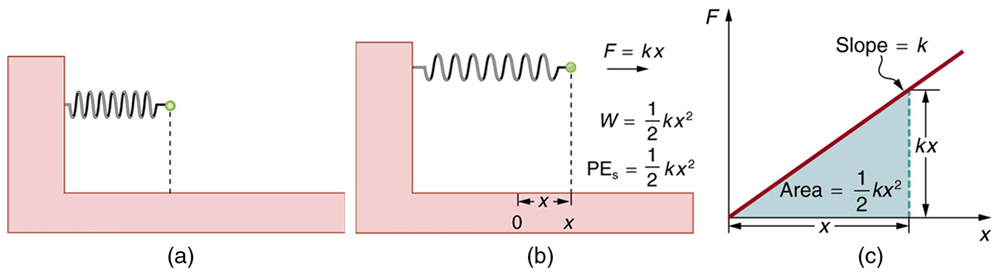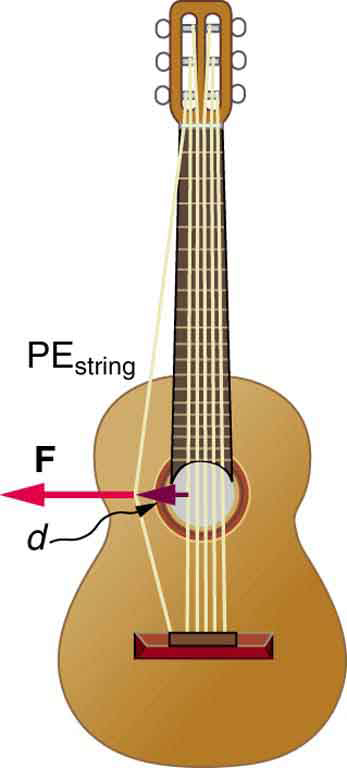# 7.4 Conservative forces and potential energy  (Page 2/8)

 Page 2 / 8
${\text{PE}}_{\text{s}}=\frac{1}{2}{\text{kx}}^{2}\text{,}$

where $k$ is the spring’s force constant and $x$ is the displacement from its undeformed position. The potential energy represents the work done on the spring and the energy stored in it as a result of stretching or compressing it a distance $x$ . The potential energy of the spring ${\text{PE}}_{s}$ does not depend on the path taken; it depends only on the stretch or squeeze $x$ in the final configuration.(a) An undeformed spring has no PE s size 12{"PE" rSub { size 8{s} } } {} stored in it. (b) The force needed to stretch (or compress) the spring a distance x size 12{x} {} has a magnitude F = kx size 12{F= ital "kx"} {} , and the work done to stretch (or compress) it is 1 2 kx 2 size 12{ { {1} over {2} } ital "kx" rSup { size 8{2} } } {} . Because the force is conservative, this work is stored as potential energy ( PE s ) size 12{ $$"PE" rSub { size 8{s} }$$ } {} in the spring, and it can be fully recovered. (c) A graph of F size 12{F} {} vs. x size 12{x} {} has a slope of k size 12{k} {} , and the area under the graph is 1 2 kx 2 size 12{ { {1} over {2} } ital "kx" rSup { size 8{2} } } {} . Thus the work done or potential energy stored is 1 2 kx 2 .

The equation ${\text{PE}}_{s}=\frac{1}{2}{\text{kx}}^{2}$ has general validity beyond the special case for which it was derived. Potential energy can be stored in any elastic medium by deforming it. Indeed, the general definition of potential energy    is energy due to position, shape, or configuration. For shape or position deformations, stored energy is ${\text{PE}}_{s}=\frac{1}{2}{\text{kx}}^{2}$ , where $k$ is the force constant of the particular system and $x$ is its deformation. Another example is seen in [link] for a guitar string.Work is done to deform the guitar string, giving it potential energy. When released, the potential energy is converted to kinetic energy and back to potential as the string oscillates back and forth. A very small fraction is dissipated as sound energy, slowly removing energy from the string.

## Conservation of mechanical energy

Let us now consider what form the work-energy theorem takes when only conservative forces are involved. This will lead us to the conservation of energy principle. The work-energy theorem states that the net work done by all forces acting on a system equals its change in kinetic energy. In equation form, this is

${W}_{\text{net}}=\frac{1}{2}{\text{mv}}^{2}-\frac{1}{2}{{\text{mv}}_{0}}^{2}=\Delta \text{KE.}$

If only conservative forces act, then

${W}_{\text{net}}={W}_{\text{c}}\text{,}$

where ${W}_{c}$ is the total work done by all conservative forces. Thus,

${W}_{\text{c}}=\text{Δ}\text{KE.}$

Now, if the conservative force, such as the gravitational force or a spring force, does work, the system loses potential energy. That is, ${W}_{\text{c}}=-\text{Δ}\text{PE}$ . Therefore,

$-\text{Δ}\text{PE}=\text{Δ}\text{KE}$

or

$\text{Δ}\text{KE}+\text{Δ}\text{PE}=0.$

This equation means that the total kinetic and potential energy is constant for any process involving only conservative forces. That is,

where i and f denote initial and final values. This equation is a form of the work-energy theorem for conservative forces; it is known as the conservation of mechanical energy    principle. Remember that this applies to the extent that all the forces are conservative, so that friction is negligible. The total kinetic plus potential energy of a system is defined to be its mechanical energy    , $\left(\text{KE}+\text{PE}\right)$ . In a system that experiences only conservative forces, there is a potential energy associated with each force, and the energy only changes form between $\text{KE}$ and the various types of $\text{PE}$ , with the total energy remaining constant.

full meaning of GPS system
how to prove that Newton's law of universal gravitation F = GmM ______ R²
sir dose it apply to the human system
prove that the centrimental force Fc= M1V² _________ r
prove that centripetal force Fc = MV² ______ r
Kaka
how lesers can transmit information
griffts bridge derivative
below me
please explain; when a glass rod is rubbed with silk, it becomes positive and the silk becomes negative- yet both attracts dust. does dust have third types of charge that is attracted to both positive and negative
what is a conductor
Timothy
hello
Timothy
below me
why below you
Timothy
no....I said below me ...... nothing below .....ok?
dust particles contains both positive and negative charge particles
Mbutene
corona charge can verify
Stephen
when pressure increases the temperature remain what?
remains the temperature
betuel
what is frequency
define precision briefly
CT scanners do not detect details smaller than about 0.5 mm. Is this limitation due to the wavelength of x rays? Explain.
hope this helps
what's critical angle
The Critical Angle Derivation So the critical angle is defined as the angle of incidence that provides an angle of refraction of 90-degrees. Make particular note that the critical angle is an angle of incidence value. For the water-air boundary, the critical angle is 48.6-degrees.
okay whatever
Chidalu
pls who can give the definition of relative density?
Temiloluwa
the ratio of the density of a substance to the density of a standard, usually water for a liquid or solid, and air for a gas.
Chidalu
What is momentum
mass ×velocity
Chidalu
it is the product of mass ×velocity of an object
Chidalu
how do I highlight a sentence]p? I select the sentence but get options like copy or web search but no highlight. tks. src
then you can edit your work anyway you want
Wat is the relationship between Instataneous velocity
Instantaneous velocity is defined as the rate of change of position for a time interval which is almost equal to zero
AstronomyByByBy Eddie UnverzagtBy OpenStaxBy OpenStaxBy OpenStaxBy OpenStaxBy Brooke DelaneyBy Robert MurphyBy David CoreyBy OpenStaxBy Anonymous User